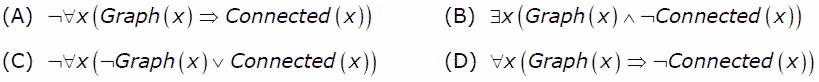# GATE | GATE-CS-2007 | Question 85

Let Graph(x) be a predicate which denotes that x is a graph. Let Connected(x) be a predicate which denotes that x is connected. Which of the following first order logic sentences DOES NOT represent the statement: “Not every graph is connected”?(A) A
(B) B
(C) C
(D) D

Explanation: Option A and option C are same G(x)–>C(x) can also be written as ~G(x) or C(x), and they are the correct representation.
Option C says :There exists a graph and graph is not connected ,which is equivalent to given sentence.
Option D: Every x which is graph is not connected.

Option D is correct choice

Quiz of this Question

My Personal Notes arrow_drop_up
Article Tags :

Be the First to upvote.

Please write to us at contribute@geeksforgeeks.org to report any issue with the above content.